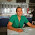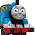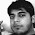## Thursday, November 28, 2013

### No. 48 - Least Number after Deleting Digits

Problem: Please get the least number after deleting k digits from the input number. For example, if the input number is 24635, the least number is 23 after deleting 3 digits.

Analysis: Let’s delete a digit from the number at each step. What’s the first digit to be deleted from the number 24635, in order to get the least number with the remaining digits? We may list all the remaining numbers after deleting a digit, in the following table:

 Deleted Digit Remaining Number 2 4635 4 2635 6 2435 3 2465 5 2463

The number 2435 is the least one in all remaining numbers, by deleting the digit 6. Notice that the digit 6 is the first digit in the number 24635 which is greater than the next digit.

Let’s delete another digit from the number 2435, the remaining least number after the first step. We may summarize the remaining numbers after delete every digit from it in the following table:

 Deleted Digit Remaining Number 2 435 4 235 3 245 5 243

The number 235 is the least one in all remaining numbers, by deleting the digit 4. Notice that the digit 4 is the first digit in the number 2435 which is greater than the next digit.

The remaining three digits in the number 235 are increasingly sorted. What is the next digit to be deleted to get the least remaining number? Again, we may list the remaining numbers after deleting each digit in a table:

 Deleted Digit Remaining Number 2 35 3 25 5 23

The number 23 is the least one in all remaining numbers, by deleting the last digit 5.

If we are going to deleting more digits from a number whose digits are increasingly sorted to get the least number, the last digit is deleted at each step.

Now we get the rules to delete digits to get the least remaining number: If there are digits who are greater than the next one, delete the first digit. If all digits in the number are increasingly sorted, delete the last digit gets deleted. The process repeats until the required k digits are deleted.

The code can be implemented in Java as the following:

public static String getLeastNumberDeletingDigits_1(String number, int k) {
String leastNumber = number;
while(k > 0 && leastNumber.length() > 0) {
int firstDecreasingDigit = getFirstDecreasing(leastNumber);
if(firstDecreasingDigit >= 0) {
leastNumber = removeDigit(leastNumber, firstDecreasingDigit);
}
else {
leastNumber = removeDigit(leastNumber, leastNumber.length() - 1);
}

--k;
}

return leastNumber;
}

private static int getFirstDecreasing(String number) {
for(int i = 0; i < number.length() - 1; ++i) {
int curDigit = number.charAt(i) - '0';
int nextDigit = number.charAt(i + 1) - '0';
if(curDigit > nextDigit) {
return i;
}
}

return -1;
}

private static String removeDigit(String number, int digitIndex) {
String result = "";
if(digitIndex > 0) {
result = number.substring(0, digitIndex);
}
if(digitIndex < number.length() - 1) {
result += number.substring(digitIndex + 1);
}

return result;
}

Optimization: Save the start index for the next round of search for the first decreasing digit

In the method getFirstDecreasing above to get the first digit which is greater than the next one, we always start from the first digit. Is it necessary to start over in every round of search?

The answer is no. If the ith digit is the first digit which is greater than the next one, all digits before the ith digit are increasingly sorted. The (i-1)th digit might be less than the (i+1)th digit, the next digit of the (i-1)th digit after the ith digit is deleted. Therefore, it is safe to start from the (i-1)th digit in the next round of search.

With this optimization strategy, the efficiency gets improved from O(n*k) to O(n), if the length of the input number has n digits and k digits are deleted.

The optimized solution can be implemented as:

class DecreasingResult {
public int firstDecreasing;
public int nextStart;
}

public static String getLeastNumberDeletingDigits_2(String number, int k) {
String leastNumber = number;
int start = 0;
while(k > 0 && leastNumber.length() > 0) {
DecreasingResult result = getNextDecreasing(leastNumber, start);
if(result.firstDecreasing >= 0) {
leastNumber = removeDigit(leastNumber, result.firstDecreasing);
}
else {
leastNumber = removeDigit(leastNumber, leastNumber.length() - 1);
}

start = result.nextStart;
--k;
}

return leastNumber;
}

private static DecreasingResult getNextDecreasing(String number, int start) {
int firstDecreasing = -1;
int nextStart;

for(int i = start; i < number.length() - 1; ++i) {
int curDigit = number.charAt(i) - '0';
int nextDigit = number.charAt(i + 1) - '0';
if(curDigit > nextDigit) {
firstDecreasing = i;
break;
}
}

if(firstDecreasing == 0) {
nextStart = 0;
}
else if (firstDecreasing > 0) {
nextStart = firstDecreasing - 1;
}
else {
nextStart = number.length();
}

DecreasingResult result = new DecreasingResult();
result.firstDecreasing = firstDecreasing;
result.nextStart = nextStart;

return result;
}

Code with unit tests is shared at http://ideone.com/0Mdfcf.

More coding interview questions are discussed in my book <Coding Interviews: Questions, Analysis & Solutions>. You may find the details of this book on Amazon.com, or Apress.

The author Harry He owns all the rights of this post. If you are going to use part of or the whole of this ariticle in your blog or webpages, please add a reference to http://codercareer.blogspot.com/. If you are going to use it in your books, please contact him via zhedahht@gmail.com . Thanks.

1.This comment has been removed by the author.

1.Hi Harry,

Three cheers to you! Hooray!!! I feel like I hit the jackpot on No. 48 - Least Number after Deleting Digits!

I logged into console.aws.amazon.com today and now every few seconds a popup says I need to reload (and re-login). This has happened before after a long time of inactivity, but now it's happening so often I can't look at anything without having to reload and login.
In Spot and On-demand instance, AWS Training USA there is no commitment for the duration from the user side, however in reserved instances, one has to stick to the time period that he has chosen.

THANK YOU!! This saved my butt today, I’m immensely grateful.

Kevin

2.This is a long method and might not work some cases. ex: 24631, this method will return 21, but the right answer should be 12.
Easy way:
1.) sort all the digits in ascending order.
2.) delete the last k digits

1.Thanks for your reply. There is some misunderstanding. The allowed operation is just to delete some digits. Reordering digits is not allowed.

2.we cannot sort digits

3.import java.util.ArrayList;
import java.util.Collections;
import java.util.List;

public class DeleteDigit {

public static List splitAndGiveList(String number){
int length=number.length();
List list=new ArrayList();
for(int i=0;i<length;i++){
String newNum=number.substring(0,i)+number.substring(i+1,length);
}
Collections.sort(list);
return list;
}

public static void findLeast(String number,int noOfDigit){
Integer leastNo=0;
for(int i=0;i<noOfDigit;i++){
leastNo=splitAndGiveList(number).get(0);
number=leastNo.toString();
}
System.out.println(leastNo);
}
/**
* @param args
*/
public static void main(String[] args) {
findLeast("24631",3);

}

}

1.Very much useful article. Kindly keep blogging

Java Training in Chennai

Java Online Training India

4.what's the reasoning behind it??

5.A ligth solution ... just take a 10 byte array for each digit, count no of appearance. Traverse the auxiliary array and compose the output number.

public static int LeastNumberAfterDeletingDigits(int inputNo, int noOfDigitsToDelete)
{
var s = inputNo.ToString();
var digits = new byte;
foreach (var ch in s)
{
digits[(byte)(ch - '0')]++;
}

var output = 0;
var cnt = s.Length - noOfDigitsToDelete;
for (int i = 0; i < digits.Length; i++)
{
for (int j = 0; j < digits[i] && cnt > 0; j++)
{
cnt--;
output = output * 10 + i;
}

if (cnt == 0) break;
}

return output;
}

1.Nice, I was thinking the same but I believe this does not take into account order.

eg:
input: ("321",1) //delete 1 digit from value 321
expected: 21
returns: 12

6.7.This comment has been removed by the author.

8.#include <iostream>

void removeLeastK(char* s, unsigned k)
{
if (!s) return;
unsigned j = 1;

for(int i = 0, deleted = 0; s[j] != '\0'; j++)
{
for(; deleted < k && i >= 0 && s[i] > s[j]; deleted++, i--);
s[++i] = s[j];
}

if (k > j) k = j;
s[j - k] = '\0';

std::cout << s << std::endl;
}

void main(void)
{
char s1[] = "24635";
removeLeastK(s1, 3);

removeLeastK(s1, 6);

char s2[] = "45532761";
removeLeastK(s2, 4);
}

9.Can't we just delete the k maximum digits?
Will it pass?

10.Simplest way is to convert number into character Array and then sort . Finally concatenate all characters till the deleteIndex.

private static int getLeastNumberAfterDelete(int num, int dighitsDel) {

String s = "";
char[] charArray = String.valueOf(num).toCharArray();
Arrays.sort(charArray);
for (int i = 0; i < (charArray.length - dighitsDel); i++) {
s += charArray[i];
}

return Integer.valueOf(s);
}

11.Hi, Harry, any formal proof for the correctness of this algorithm could be provided. How can we know that it reaches the global least number by making it the least number after deleting one digit at each step. Thanks for the solution, it's very nice.

12.This algorithm is not correct for the case getLeastNumberDeletingDigits_1("21463", 3), which outputs 13 instead of 21.

13.This comment has been removed by the author.

14.Find largest k elements in the number, and store them in a set .Can be done in O(n) and requires O(k) space.Then O(n) to remove all the numbers in the set.

15.Hi There,

Brilliant article, glad I slogged through the No. 48 - Least Number after Deleting Digits it seems that a whole lot of the details really come back to from my past project.

Currently retrieving account attributes
We are currently in the process of retrieving your account attributes . Please try again in a few minutes.

I am getting this error message for the last couple of days. According to it is about my account not having been activated. But according to am email from Amazon, my account is fully activated.

Anyways great write up, your efforts are much appreciated.

Preethi.

16.Szia,

Hot! That was HOT! Glued to the No. 48 - Least Number after Deleting Digits your proficiency and style!

We have a customer in Denmark whose legal department is asking for the physical address of the data center in which their data is being processed. The region/AZ in question would be eu-west-1a+b. Is there any chance AWS will disclose the address for this purpose? AWS Tutorial

I read multiple articles and watched many videos about how to use this tool - and was still confused! Your instructions were easy to understand and made the process simple.

Thanks,
17.18.